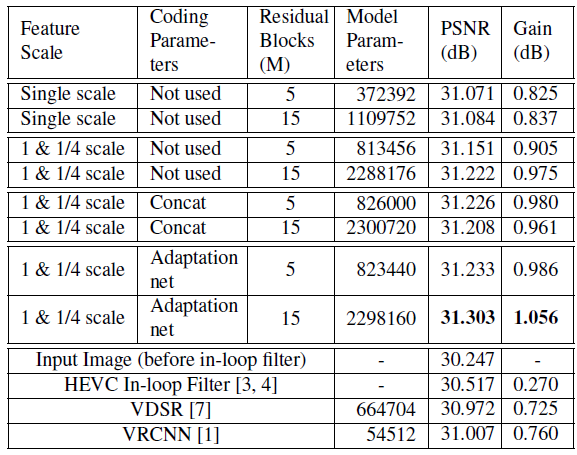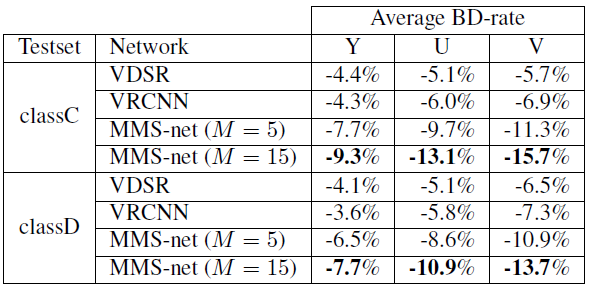# Review: MMS-net — Multi-Modal/Multi-Scale Convolutional Neural Network (Codec Filtering)

## Outperforms VDSR and VRCNN, Average 8.5 % of BD-Rate Reduction is Obtained

In this paper, Multi-Modal/Multi-Scale Convolutional Neural Network (MMS-net), by Seoul National University and Korea Electronics Technology Institute, is reviewed. By inputting the image into two sub-networks for different scales, with additional coding parameter (CP) information, MMS-net outperforms VDSR and VRCNN. This is a paper in 2017 ICIP. (Sik-Ho Tsang @ Medium)

# Outline

1. Coding Parameters (CP) Format
2. MMS-net Network Architecture
3. Experimental Results

# 1. Coding Parameters (CP) Format

• Blocking artifacts are mainly caused by block-based compression mechanism. Thus, given the block partitioning information, the network can easily locate and eliminate blocking artifacts.
• In the HEVC standard, CTU is the basic processing unit for compression. A CTU is divided into quadtrees of coding units (CUs) recursively, and each CU is composed of predictive units (PUs) and transformation units (TUs).
• (If interested, please visit Sections 1 & 2 in IPCNN for what video coding is. For the sake of simplicity, PU and TU are the further partitions under CU for more efficient compression.)
• Then, the value of ‘2’ is assigned to the positions corresponding to the outermost pixels of each CU and TU, and the value of ‘1’ is set for the non-boarder area in each matrix.
• Therefore, two matrices of the input size are generated for each frame. One for CU and one for TU.

# 2. MMS-net Network Architecture

## 2.1. Adaptation Network

• A simple pre-network (called adaptation network) that transforms CP information space into image feature space.
• A combination of convolution layers and ReLU layers projects the CP matrices into a single channel feature map.
• Then, the CP feature map is element-wisely multiplied with the input image and concatenated as an additional channel to the input image.
• The structure of the adaptation network is shown in the upper left corner of the above figure.

## 2.2. Multi-Scale Sub-networks

• Multi-scale image restoration architecture has been utilized in various image restoration researches.
• It can be viewed as a hierarchical process in a multi-scale image space so that the restored image retains small details on finer scales as well as long-range dependencies on coarser scales.
• The concept of coarse-to-fine restoration has proven useful in sharpening severely blurred images.
• In this paper, the proposed model has 2 sequential sub-networks of different scales (K = 2).
• Instead of resizing the image before inputting into the network, a convolutional layer with stride 2 is for down-sampling and a deconvolutional layer is for up-sampling.
• The sub-network structure of each scale is a modified version of the SRResNet. (I hope I can review this in the coming future.)
• The basic building unit of the network is residual blocks originated in ResNet.
• Each residual block contains two consecutive sub-modules consisting of a batch normalization layer, a ReLU layer, and a convolution layer.
• A global skip connection is also used.
• M number of residual blocks for each scale path.
• All convolutional layers in the network use 3×3 kernels, except for the first convolutional layer of each scale’s sub-network which uses 7 × 7 kernels.
• No fully connected layers in the network.

## 2.3. Multi-Scale Loss

• The loss on each sub-network is computed, summed ad averaged:
• R_k, G, and K denote the output of the k-th sub-network, a ground truth image, and the number of sub-networks (scales), respectively. The loss is normalized by width w and height h of the input image.
• 28 HD sequences in YCbCr 420 color format from Xiph.org Video Test Media are used for training.
• Samples are obtained by encoding the training sequences using HM-16.7.
• Ground-truth are the original sequences.

# 3. Experimental Results

## 3.1. PSNRPSNRs Obtained by Different Configurations and SOTA Approaches
• Evaluation is done on all sequences in class D.
• As shown above, multi-scale network improves the performance by 0.08–0.14 dB.
• Concatenating CP images along channel axis of an input image is helpful in the 5 residual-block network, but makes worse for the 15 residual-block network.
• However, a gain of 0.08 dB is obtained through the CP adaptation network (pre-network) for both the 5 and 15 residual-block networks.
• VDSR has a larger number of variables than VRCNN, it fails to find a better optimal point and performance is worse than VRCNN.
• And MMS-net outperforms both VDSR and VRCNN.

## 3.2. BD-Rate (Bitrate)BD-rate (%) Against the conventional HEVC HM-16.7
• MMS-net (M = 15) achieves the superior performance by reducing average 8.5 % of BD-rate for the Y channel over the HEVC baseline.
• This model is trained only on the Y channel, but further reduces the BD-rate on the U and V channels.

## 3.3. Subjective Quality

• The above results demonstrate that the proposed MMS-net effectively removes the blocking artifacts while preserving major contents and sharp edges of an image.

# Codec Filtering

[ARCNN] [Lin DCC’16] [IFCNN] [Li ICME’17] [VRCNN] [DCAD] [MMS-net] [DRN] [Lee ICCE’18] [DS-CNN] [Lu CVPRW’19] [Wang APSIPA ASC’19] [RHCNN]

--

--